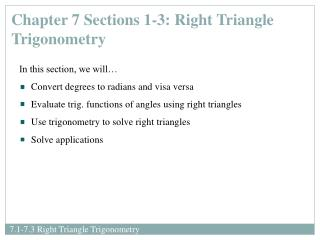DownloadDownload PresentationChapter 7 Sections 1-3: Right Triangle Trigonometry

# Chapter 7 Sections 1-3: Right Triangle Trigonometry

Download Presentation## Chapter 7 Sections 1-3: Right Triangle Trigonometry

- - - - - - - - - - - - - - - - - - - - - - - - - - - E N D - - - - - - - - - - - - - - - - - - - - - - - - - - -
##### Presentation Transcript

1. Chapter 7 Sections 1-3: Right Triangle Trigonometry • In this section, we will… • Convert degrees to radians and visa versa • Evaluate trig. functions of angles using right triangles • Use trigonometry to solve right triangles • Solve applications

2. There are two common ways to measure angles, in degrees and in radians. • We’ll start with degrees, denoted by the symbol º. • A second way to measure angles is in radians... One degree (1º) is equivalent to a rotation of of one revolution. One radian is the measure of a central angle  that intercepts arc s equal in length to the radius r of the circle. arc length = radius when 1 radian

3. To convert degrees to radians, multiply degrees by Examples:Convert from degrees to radians: 210º Convert from degrees to radians: -675º To convert radians to degrees, multiply degrees by Examples:Convert from radians to degrees: Convert from radians to degrees:

4. Hypotenuse Side opposite  Side adjacent to  The sides of a right triangle, with an acute angle , are labeled in reference to . • We will be reviewing special ratios of these sides of the right triangle, with respect to angle, . • These ratios are better known as our six basic trig functions: • sine • cosine • tangent • cosecant • secant • cotangent

5. To remember the definitions of sine, cosine and tangent, we use the acronym : “SOH CAHTOA”

6. 5 3 Example:Find the value of each of the six trigonometric functions of the angle .

7. 3 6 Example:Find the value of each of the six trigonometric functions of the angle .

8. Example: Given , find the value of each of the four remaining trigonometric functions of

9. 45º 1 1 Special Right Triangles: the 45º- 45º- 90º triangle ratio of sides: Find the exact values of the six trig functions for 45 sin 45 = csc 45 = cos 45 = sec 45 = tan 45 = cot 45 = 45º IMPORTANT TO MEMORIZE FOR MAT204!

10. 30º 2 60º 1 Special Right Triangles: the 30º- 60º- 90º triangle ratio of sides: Find the exact values of the six trig functions for 30 sin 30 = csc30 = cos30 = sec 30 = tan 30 = cot 30 = IMPORTANT TO MEMORIZE FOR MAT204!

11. 30º 2 60º 1 Special Right Triangles: the 30º- 60º- 90º triangle ratio of sides: Find the exact values of the six trig functions for 60 sin 60 = csc 60 = cos 60 = sec 60 = tan 60 = cot 60 = IMPORTANT TO MEMORIZE FOR MAT204!

12. To evaluate trig functions of acute anglesother than 30, 45, and 60, use TI. • Make sure the MODE is set to the correct unit of angle measure. • (Degree vs. Radian) • Example: Findtan 50 degrees to two decimal places. • Solving Right Triangles • When you use the given information to find all three of the angle measure and all three of the side-lengths, you will use a process called solving right triangles. • Sketch a right triangle and label the angle and/or side(s) given • Use the definitions of the six trig functions to determine which function is appropriate to use. • If you have angles of 30º, 60º, or 45º, use the special reference triangles (ratios of the sides) to solve the triangle. Otherwise, use your TI to evaluate.

13. 52º 9.6 Example:Solve the given right triangle. note

14. Example:

15. Example:

16. Independent Practice You learn math by doing math. The best way to learn math is to practice, practice, practice. The assigned homework examples provide you with an opportunity to practice. Be sure to complete every assigned problem (or more if you need additional practice). Check your answers to the odd-numbered problems in the back of the text to see whether you have correctly solved each problem; rework all problems that are incorrect. Read pp. 504-513 and 517-525 Homework: pp. 514 #35-57 odds pp. 526 #11, 15-31 odds pp.536-539 #5, 6, 55, 59, 61, 63